# Explainability for tree-based models: which SHAP approximation is best?

By Giovanni Baj and Giovanni Cinà (Pacmed Labs)

In a nutshell. At Pacmed we care about improving medical practice with the help of Artificial Intelligence (AI). We often use tree-based models, and employ SHAP values to understand what the models do. But which SHAP approximation is best to use? In this post we share our findings.

# Introduction

AI models have a huge potential to improve healthcare quality and drive personalized medicine, but their adoption in clinical practice is still very limited. One of the key barriers to the implementation of AI is the lack of transparency of its algorithms¹. There are plenty of reasons why a certain level of transparency is needed when applying AI in the medical domain, including:

• build users’ trust: as clinicians are the ones responsible to give the best care to each patient, they should be confident that AI technologies can be trusted, and to make it possible they need to know how the model arrived to a certain prediction
• justify decisions and comply with the ‘right to explanation’: it is important for clinicians to be able to justify their decision making towards their patients and colleagues
• detect biases: models interpretability would enable examination for any potential bias (e.g. discrimination based on race, sex or other sensible features)

Most performant models available now, such as ensembles or deep learning models, are very complex and hard to interpret. Therefore, what we need are systematic methods to make these models transparent and allow humans to understand how a certain prediction was reached. One of the most prominent tools for this purpose is the SHAP method, introduced by Lundberg and Lee in 2017. SHAP’s basic intuition is very simple, and it consists in estimating how much each feature contributes to the outcome of a single prediction.

In the case of tabular data, machine learning models based on trees are some of the most popular and powerful models in use today. To explain this kind of models using SHAP framework, two different algorithms, known as TreeSHAP algorithms, were developed. Which one is best to use? The goal of this article is to show some of the differences between the two algorithms and their potential failure modes.

# What are SHAP values?

SHAP (SHapley Additive exPlanations) is a game theoretic approach to explain the output of any machine learning model. As we have already mentioned, SHAP method attributes to each feature an importance value (named SHAP value) that represents the contribution of that feature to the final outcome of the model. Suppose for example that we have a model f(x) and that we want to explain the prediction of the model on a specific sample x*. To do so, SHAP method decomposes the prediction into the sum of the contributions of all the features, namely

where 𝜙₀ is the average model’s prediction. To compute each contribution 𝜙ⱼ, SHAP method relies on a concept that comes from cooperative game theory, known as Shapley value, which is defined as the average marginal contribution of a feature value over all possible coalitions. Translating this definition in formulas, the Shapley value of feature i becomes

where the sum is performed over all the possible subsets of features that do not contain feature i and 𝑓ₓ(S) is the prediction of the model when only features in S are known. In this way, the terms [𝑓ₓ(S U {i}) - 𝑓ₓ(S)] that we are summing are the marginal contributions of the feature to all the coalitions of features.

The first thing that should be noticed about the equation above is that we have to perform a sum over all the possible subsets of features. Since the number of subsets grows exponentially with the number of features, it is clear that it is computationally impossible to compute Shapley values applying directly the definition. Therefore we need efficient algorithms to compute good approximations of SHAP values. In the case of tree ensemble models, two algorithms (named TreeSHAP algorithms) were specifically designed to explain this type of models in a very efficient way.

The second important detail of the equation that defines Shapley values is that to compute 𝑓ₓ(S) we should in principle evaluate the model only on a subset of features. Notice, however, that this is not possible for a generic ML model, since we need to evaluate it on all the input features. So the problem is how to “drop” the features that are not in the subset S, keeping in mind that 𝑓ₓ(S) should represent the prediction of the model when we know only the features in S. There are two possible approaches:

• Observational: The first possibility is to use the expected output of the predictive model conditional on the feature values of the subset S for sample x*
• Interventional: The second possibility is to break the correlation between features and compute the marginal expectation value of the model

Of course, both methods have advantages and disadvantages. For example observational Shapley values suffer from two problems: 1) they can give importance also to features not used by the model, and 2) they can spread importance between correlated features. On the other hand, if we use an interventional approach, we are assuming that features are not correlated, and in this way we are evaluating the model on (potentially) impossible data-points and this can give rise to unreliable explanations.

Now you may wonder which of the two approaches should be used, but actually there is no general agreement on this point in the literature. Some papers² assert that the interventional approach is the one that should be adopted, other papers³ claim the exact opposite, while still other papers⁴ suggest that the correct approach depends on the specific application. As you can imagine, the problem is quite relevant, since the choice of one approach or the other can lead to different results.

The distinction between observational and interventional algorithms is very important when we use TreeSHAP method, since also the two available algorithms follow this distinction. More precisely, the two algorithms are:

• tree_path_dependent TreeSHAP: it is supposed to observational. Why “supposed”? Well, in the paper⁵ where it was introduced the authors claimed that it was observational, but we will see that it does not have many properties typical of observational approaches.
• interventional TreeSHAP: it ignores feature correlation.

Which algorithm should we use then? It’s not easy to answer this question in general and with the experiments presented in the following section we will study some relevant aspects of the two algorithms that could help with deciding.

# Experiments

Questions to be answered about tree_path_dependent TreeSHAP:

• How does it deal with irrelevant features?
• Does it spread importance among correlated features?
• In which scenarios does it differ from interventional TreeSHAP?

We performed all the experiments in Python, where the package shap allows to compute SHAP values in a straightforward way. To use the TreeSHAP method one has to rely on the class `shap.explainers.Tree`. Then, the choice between the two TreeSHAP algorithms can be easily done setting the parameter `feature_perturbation`, as described in the package documentation.

## How does TreeSHAP treat irrelevant features?

One of the most contested downsides of observational methods is that they can attribute importance to irrelevant features, i.e. features which are not used by the model² ⁶. Is tree_path_dependent TreeSHAP affected by this issue?

The experiment we implemented to answer this question is the one proposed by Janzing et al², where we have two binary features X₁ and X₂ perfectly correlated and a model that gives its output only based on the first of the two features:

with the following data distribution

It is clear that when we compute the importance of the two features, we expect that only feature x₁ takes importance. On the other hand, it is shown in the original paper that if we compute analytically the observational Shapley values we obtain an importance for x₂ which is different from zero.

We implemented this same experiment in Python:

1. we generated a data-set with only two binary features perfectly correlated
2. we trained a decision tree that uses only feature x₁ to give the prediction
3. we explained the model’s predictions with tree_path_dependent TreeSHAP.

What we have found is that feature x₂ does not take any importance, as you can see in the SHAP summary plot reported here.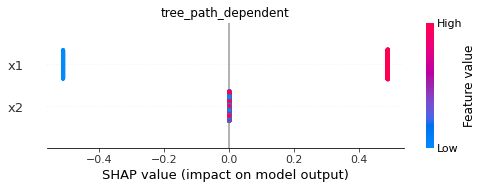SHAP summary plot for a model in which feature x₂ is irrelevant. For a fixed feature, each dot represents a datapoint, and the x-axis position corresponds to the SHAP values assigned to the feature for that datapoint. Each dot is colored based on the the feature value. As we can see x₂ takes zero importance for all the data-points.

We then repeated the same experiment, but this time using a different explanation algorithm, which this time was “truly” observational (the algorithm used is the observational version of KernelSHAP proposed by Aas et al. in 2021). Below we can see that in this case we obtain the expected result, in which also feature x₂ takes importance.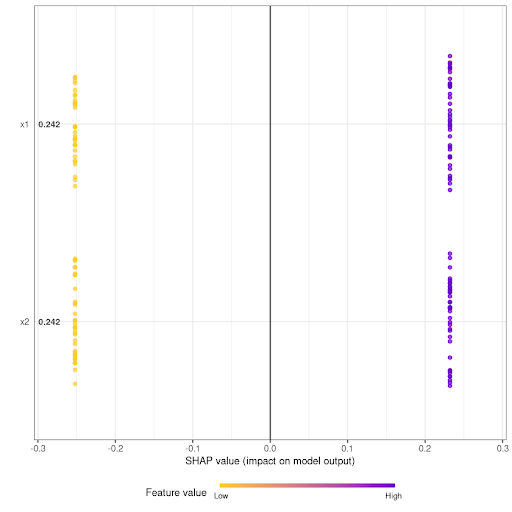SHAP summary plot for a model in which feature x₂ is irrelevant, explained with a truly observational method. This time also the second feature takes some importance.

These results are telling us that tree_path_dependent TreeSHAP is not observational from this point of view, since it does not give importance to irrelevant features. This behavior is also confirmed by an inspection of the algorithm itself.

## Algorithms comparison when features are correlated

The second experiment we performed was to compare the two TreeSHAP algorithms in a scenario where in principle there could be differences between their explanations, which is when there is relevant correlation between features that are not irrelevant.

The experiment we performed is the following:

1. a dataset of 3 features is sampled from a 3D Gaussian distribution where the last two features have a degree of linear correlation equal to
2. a binary target variable is defined from a linear combination of the predictors: 𝑦 = sign(ax₁+bx₂+ cx₃)
3. a gradient boosting classifier is trained based on this data
4. the model is explained using TreeSHAP algorithms

What we could expect in principle is that when we have high values of correlation between features the two algorithms could give significantly different explanations of the same model. In particular, we know that observational methods could spread importance among correlated features. However, we find that the two algorithms give very similar SHAP values, no matter the value of . In the following we report an example with ⍴=0.7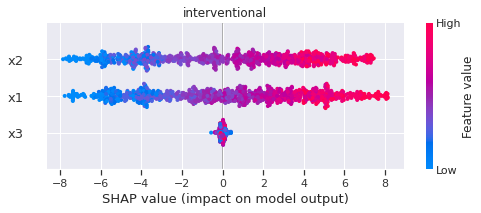SHAP summary plot for SHAP values computed with “interventional” TreeSHAP algorithm.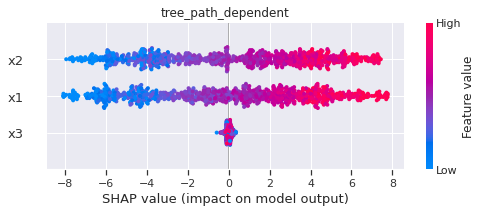SHAP summary plot for SHAP values computed with “tree_path_dependent” TreeSHAP algorithm. There are no significant differences with the “interventional” summary plot.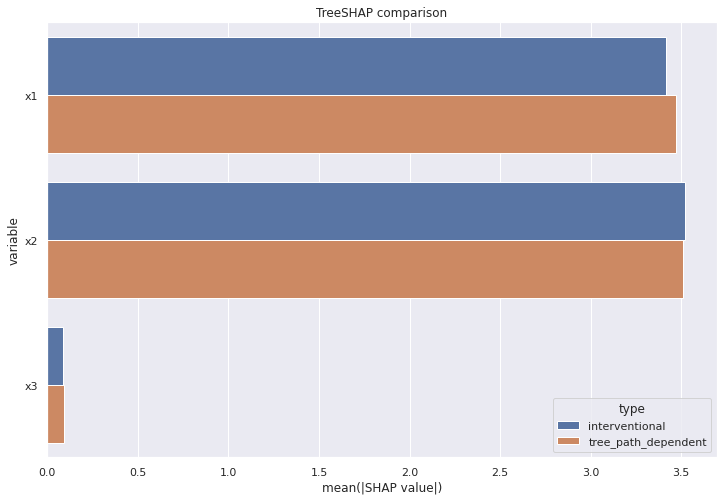Mean absolute values of SHAP values for the 3 features. As expected from the previous two plots, there are no relevant differences between the two algorithms, no matter the value of correlation.

As before, we repeated the same experiment, this time explaining the model with an algorithm that is explicitly observational (the same mentioned above). In this case we can see a clear spread of importance between correlated features, as shown below.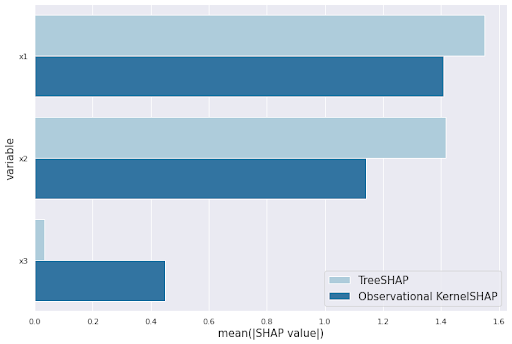Mean absolute values of SHAP values for the 3 features, computed with “tree_path_dependent” TreeSHAP and observational KernelSHAP. The observational algorithm is characterized by a spread of importance among correlated features.

Therefore, also from this point of view tree_path_dependent TreeSHAP does not behave like an observational algorithm, since it doesn’t spread at all the importance between correlated features.

## Algorithms comparison on local explanations

The comparison between the two algorithms that we have proposed in the previous section was from a “global” point of view. In this last experiment we check if the two algorithms behave differently on local explanations, since computing Shapley values for all the samples in the dataset and then average their absolute values implies losing a lot information.

We perform an experiment very similar to the previous one, with the difference that this time we have a regression problem:

1. 𝒙 is sampled from a 3D multivariate Gaussian distribution. There is a correlation between x₀ and x
2. the target 𝑦 is computed with the decision tree rule reported below
3. a decision tree to predict 𝑦 from 𝒙 is trained and then explained with TreeSHAP (notice that the decision tree model obtained after training is equal to the schema used to generate the target, as one would expect)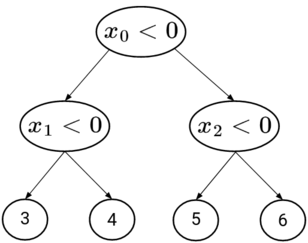Decision-tree rule to compute the target y from predictors x.

To understand in detail the behavior of the two algorithms as correlations varies, we computed, for a single fixed sample, the SHAP value of each feature as a function of ⍴. The most important results that we obtain is that tree_path_dependent TreeSHAP is influenced by features correlation, but not in the way we were expecting. More precisely, we noticed that when two features are correlated, the one that is located higher in the tree is the one that gets more importance. This effect increases with correlation degree. For example, look at the plots of sample x=[-1,-1,-1] shown below: we can see that as correlation increases, feature x₁, which is located below x₀, gets lower and lower importance (in absolute value). Conversely, increasing feature x₀ gets more and more importance.
As regards interventional SHAP values, this effect is absent since the importance of both x₀ and x₁ decreases with correlation, and this could be due to the fact that, as feature correlation changes, so does the average model’s prediction (the baseline), and SHAP values change accordingly.

# Conclusions

Explainable AI is often a requirement if we want to apply ML algorithms in high-stakes domains like the medical one. A widely used method to explain tree-based models is the TreeSHAP method, which comprises two algorithms. In this article we have presented some experiments to study the behavior and the differences between the two. The most important results are:

• (the good news) tree_path_dependent TreeSHAP is not observational strictly speaking, since it does not give importance to irrelevant features and it does not spread importance among correlated features
• (the bad news) tree_path_dependent TreeSHAP, when there is correlation between features, tends to give more importance to early-splitting features, penalizing downstream features

This behavior should be kept in mind when tree_path_dependent TreeSHAP is used to investigate the inner workings of tree models. While this algorithm does not seem to share the failure modes of other observational algorithms, one has to be mindful that the feature importance might be unreliable due to the second finding; shuffling the feature list or sampling subsets of features when building tree ensembles could be two effective ways to mitigate this problem.

References

: A. F. Markus et al, “The role of explainability in creating trustworthy artificial intelligence for health care: A comprehensive survey of the terminology, design choices, and evaluation strategies”, Journal of Biomedical Informatics (2021)

: D. Janzing et al, “Feature relevance quantification in explainable AI: A causal problem”, International Conference on Artificial Intelligence and Statistics (2020)

: C. Frye et al, “Shapley explainability on the data manifold”, ICLR (2021)

: H. Chen et al, “True to the Model or True to the Data?”, arXiv preprint arXiv:2006.16234 (2020)

: S. Lundberg et al, “Consistent Individualized Feature Attribution for Tree Ensembles”, arXiv:1802.03888 (2018)

: M. Sundararajan and A.Najmi, “The many Shapley values for model explanation”, PMLR (2020)

--

--## Pacmed

Pacmed builds decision support tools for doctors based on machine learning that makes sure patients only receive care that has proven to work for them!